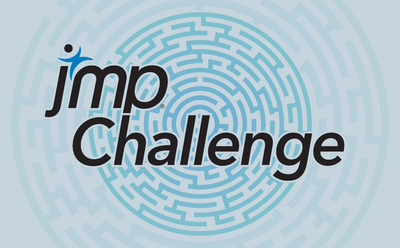Choose Language Hide Translation Bar
Challenge 2This month’s challenge is back to basics – finding prime numbers.

I am interested in two functions:

1. getPrimes(n,t) – where n is a positive integer and t can be one of two values. If it is 1 getPrimes returns the first n primes. If it is 2 getPrimes will return all primes less than or equal to n. You can assume the value of n will not exceed 1e8.
2. isPrime(vec) – where vec is a nRow row, 1 column matrix of integer values no greater than 4e14. It is to return a matrix of 1’s and 0’s where vec[i] = 1 indicates the ith element of vec is prime and 0 it is not prime. The input test vector will contain between 100 and 1000 elements (but the function should be able to accepts larger or smaller vectors).

Examples:

getPrimes(10,1) would return [2,3,5,7,11,13,17,19,23,29]

getPrimes(10,2) would return [2,3,5,7]

isPrime([-1,0,2,4,5]) would return [0,0,1,0,1]

Scoring: 4 points for each function. 3, 2, and 1 point for the fastest solution (awarded separately for each function). 3 points if your solution is as fast or faster than the fastest submitted by a staff member.

Submission deadline is Friday. May 15, 11:59 pm Eastern Time.

Article Labels
Article TagsSuper User

Thanks Don for the fun challenge. I remember when you were at our JMP Day event a few years back we did something similar to Challenge 1.

Cheers, LandonStaff

@landon I remember it, too and it definitely influenced the first challenge.

Level VI

for getPrimes(), the "assume  n will not exceed 1e8", is the for both t=1 and t=2?  I ask because I think finding the first 100 million primes might be a stretch for JMP.Staff

@MathStatChem - yes, the limit is for both t=1 and t=2 is 1e8. The value I ultimately use for testing will depend on how quickly the submitted scripts run.

Level VI

Nice website to use to check your results:  https://primes.utm.edu/nthprime/index.php

Level III

How exactly do we post a solution? Just as a comment to this post?Staff

@DavidLeber it's best if you send me the solution via email or private message on the Community. After the 15th, I'll have another post talking about the solutions.